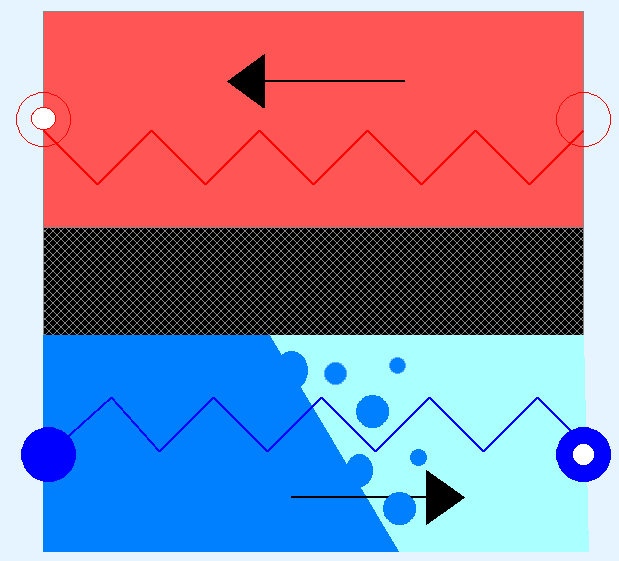# Moving Boundaries Heat Exchanger

Counter current Moving Boundary model: Fluid enters subcooled and exits in super-heated conditions. The model consider the fluid in one side and the metal wall. The secondary fluid is a constant specific heat fluid# Information

Model MBeva is a moving boundaries heat exchanger model for evaporators. The model considers a fictitious heat transfer channel split up into three different section based on the phase state of the working fluid:

• Sub-cooled zone (SB)
• Two-phase zone (TP)
• SuperHeated zone (SH)

Pressure and enthalpy are selected as state variables for each of the three zones.

The name moving boundary is derived from the fact that the interfaces between these sections do not have a fixed spatial position but merely a fixed thermodynamic location depending on the presence of liquid and gaseous fluid, respectively. The actual existence of a certain section and its length are determined based on the fluid state resulting in variable sectioning. A fixed total length superimposes the required boundary condition to calculate the length of each section.

The assumptions for this model are:

• The tube is cylindrical with a constant cross sectional area
• The velocity of the fluid is uniform on the cross sectional area
• The enthalpy of the fluid is linear in each region of the tube (sub-cooled, two-phase, super-heated)
• Pressure is considered constant
• Thermal energy accumulation in the metal wall is taken into account
• The secondary fluid is treated as a constant heat capacity fluid
• The heat transfer between the secondary fluid and the metal wall and between the metal wall and the primary fluid is computed using the epsilon-NTU method